# R S Aggarwal Solutions for Class 11 Maths Chapter 30 Statistics Exercise 30D

R S Aggarwal Solutions for chapter – Statistics will help students to understand the steps involved in the comparison of two frequency distributions with the same mean. In this exercise, students will learn how to find coefficient of variance with the help of various solved examples. R S Aggarwal Class 11 Chapter 30 Solutions are outlined and solved by the experts at BYJU’S.

Class 11 Maths Chapter 30 Statistics Exercise 30D is based on the following topics:

• Coefficient of variance
• Comparison of two frequency distributions with the same mean

## Download PDF of R S Aggarwal Solutions for Class 11 Maths Chapter 30 Statistics Exercise 30D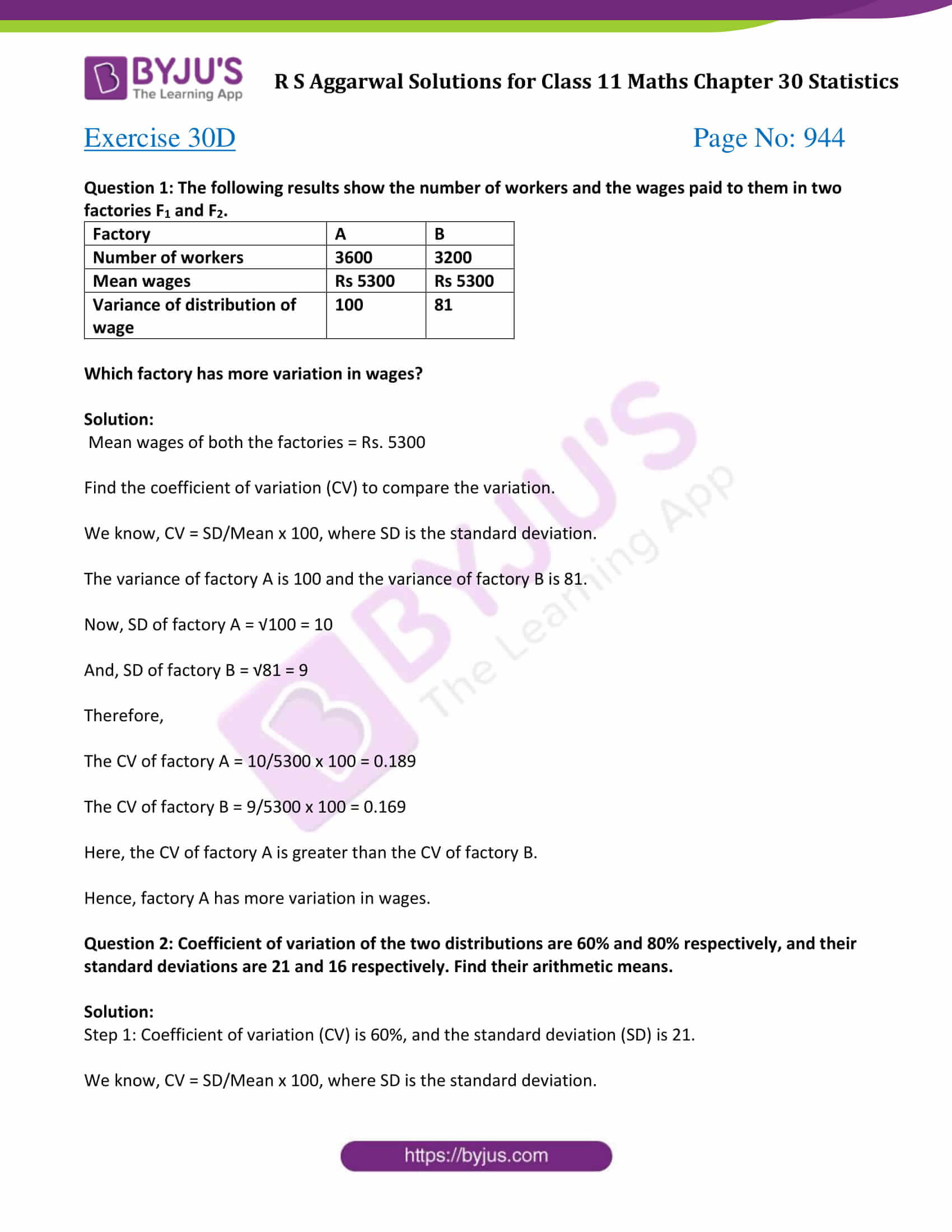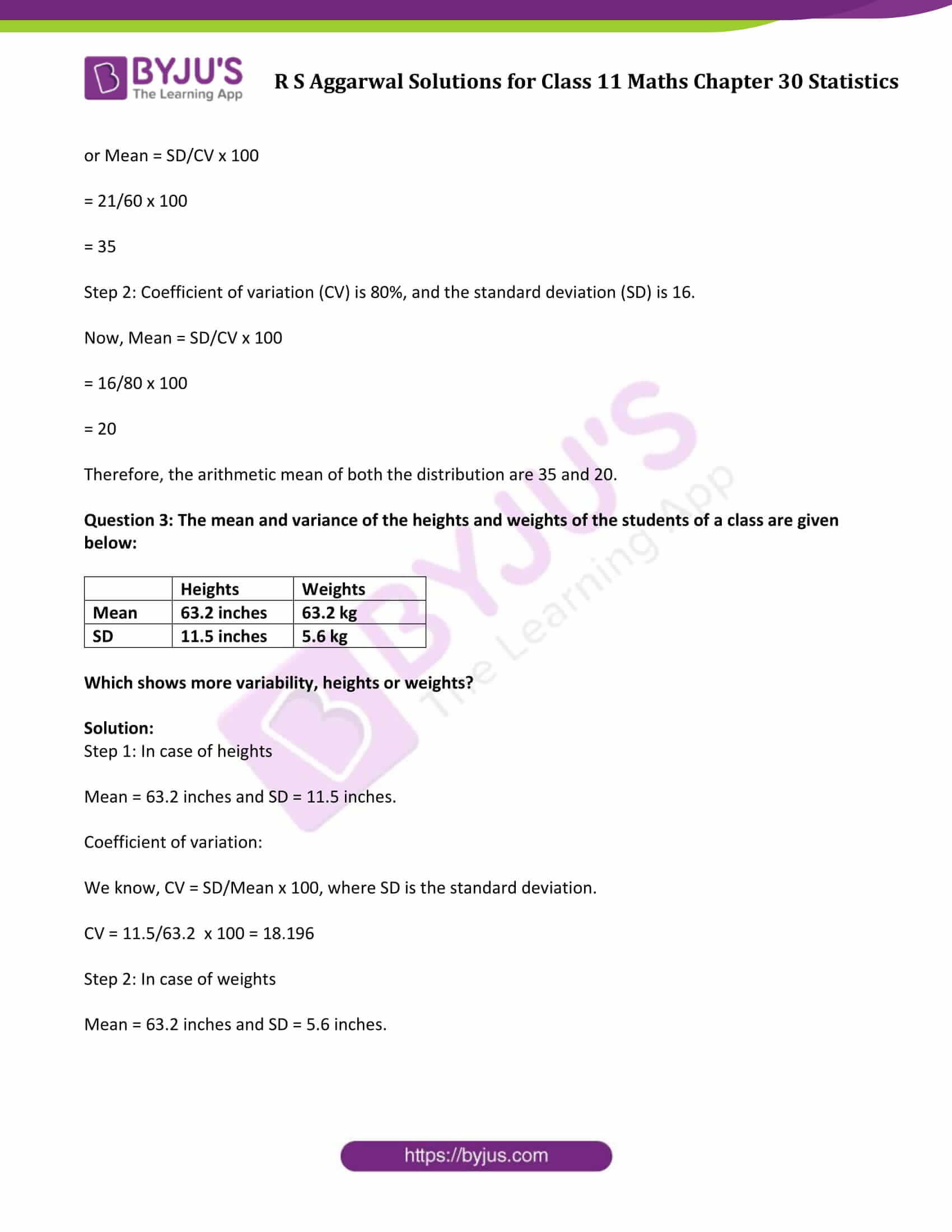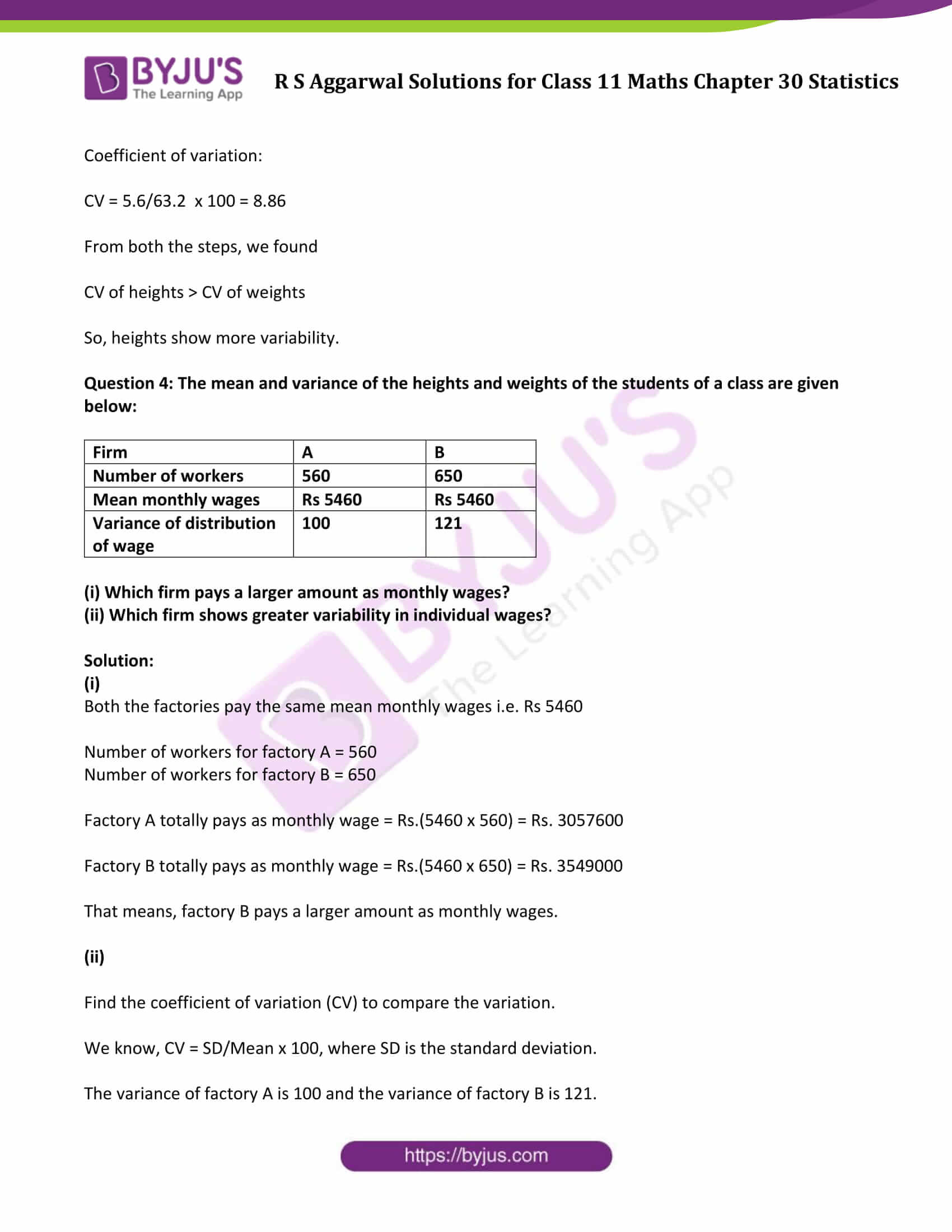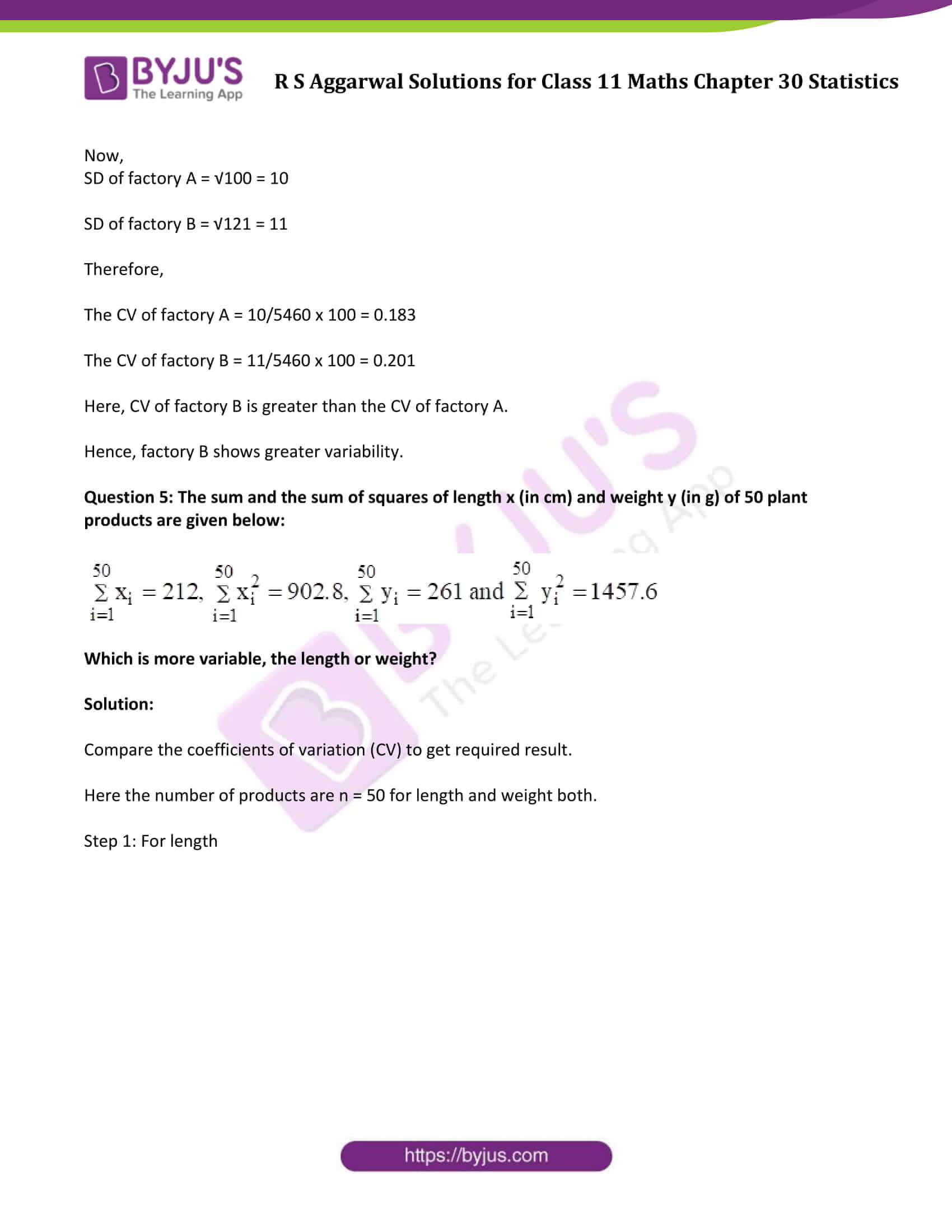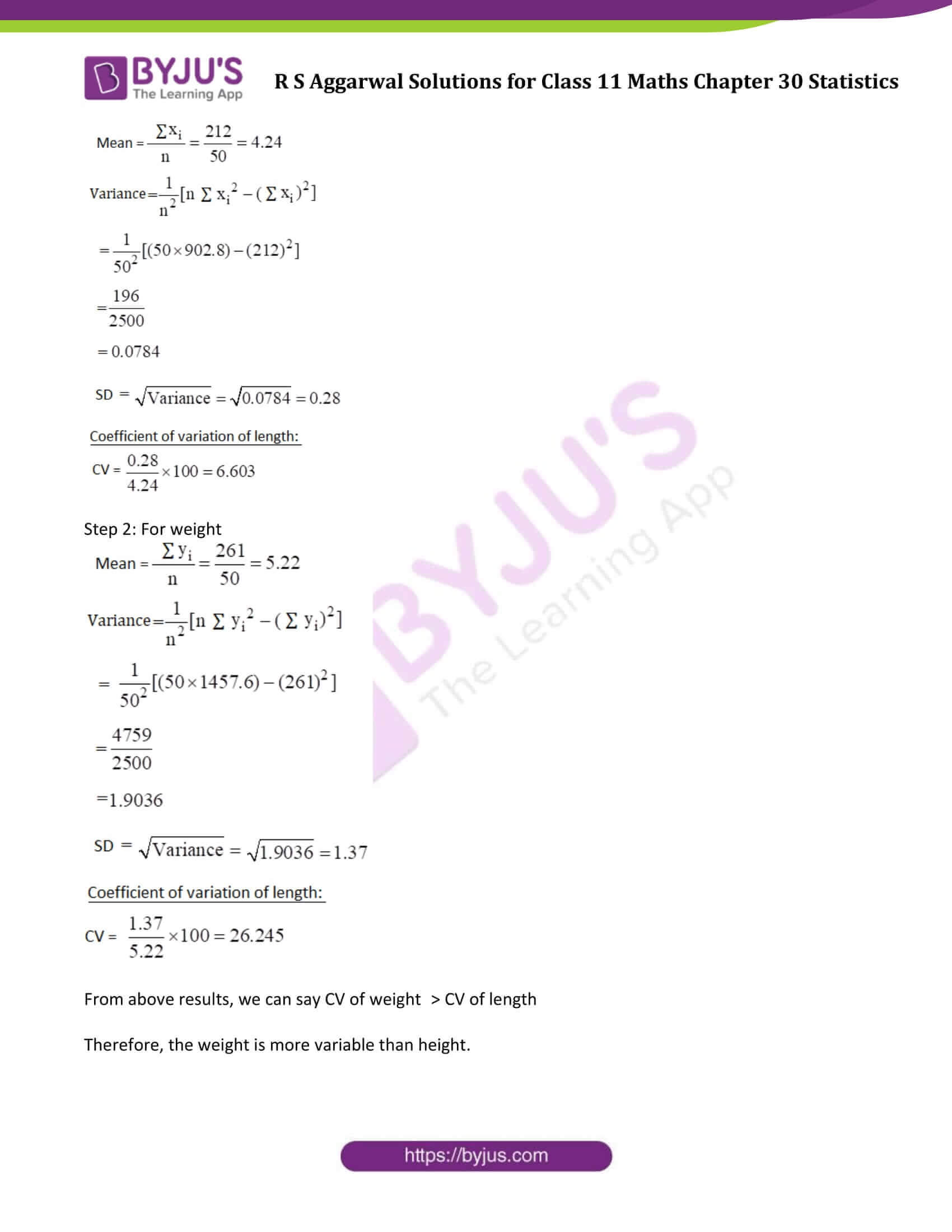### Access Answers to Maths R S Aggarwal Class 11 Chapter 30 Statistics Exercise 30D Page number 944

Question 1: The following results show the number of workers and the wages paid to them in two factories F1 and F2.

 Factory A B Number of workers 3600 3200 Mean wages Rs 5300 Rs 5300 Variance of distribution of wage 100 81

Which factory has more variation in wages?

Solution:

Mean wages of both the factories = Rs. 5300

Find the coefficient of variation (CV) to compare the variation.

We know, CV = SD/Mean x 100, where SD is the standard deviation.

The variance of factory A is 100 and the variance of factory B is 81.

Now, SD of factory A = √100 = 10

And, SD of factory B = √81 = 9

Therefore,

The CV of factory A = 10/5300 x 100 = 0.189

The CV of factory B = 9/5300 x 100 = 0.169

Here, the CV of factory A is greater than the CV of factory B.

Hence, factory A has more variation in wages.

Question 2: Coefficient of variation of the two distributions are 60% and 80% respectively, and their standard deviations are 21 and 16 respectively. Find their arithmetic means.

Solution:

Step 1: Coefficient of variation (CV) is 60%, and the standard deviation (SD) is 21.

We know, CV = SD/Mean x 100, where SD is the standard deviation.

or Mean = SD/CV x 100

= 21/60 x 100

= 35

Step 2: Coefficient of variation (CV) is 80%, and the standard deviation (SD) is 16.

Now, Mean = SD/CV x 100

= 16/80 x 100

= 20

Therefore, the arithmetic mean of both the distribution are 35 and 20.

Question 3: The mean and variance of the heights and weights of the students of a class are given below:

 Heights Weights Mean 63.2 inches 63.2 kg SD 11.5 inches 5.6 kg

Which shows more variability, heights or weights?

Solution:

Step 1: In case of heights

Mean = 63.2 inches and SD = 11.5 inches.

Coefficient of variation:

We know, CV = SD/Mean x 100, where SD is the standard deviation.

CV = 11.5/63.2 x 100 = 18.196

Step 2: In case of weights

Mean = 63.2 inches and SD = 5.6 inches.

Coefficient of variation:

CV = 5.6/63.2 x 100 = 8.86

From both the steps, we found

CV of heights > CV of weights

Question 4: The mean and variance of the heights and weights of the students of a class are given below:

 Firm A B Number of workers 560 650 Mean monthly wages Rs 5460 Rs 5460 Variance of distribution of wage 100 121

(i) Which firm pays a larger amount as monthly wages?

(ii) Which firm shows greater variability in individual wages?

Solution:

(i)

Both the factories pay the same mean monthly wages i.e. Rs 5460

Number of workers for factory A = 560

Number of workers for factory B = 650

Factory A totally pays as monthly wage = Rs.(5460 x 560) = Rs. 3057600

Factory B totally pays as monthly wage = Rs.(5460 x 650) = Rs. 3549000

That means, factory B pays a larger amount as monthly wages.

(ii)

Find the coefficient of variation (CV) to compare the variation.

We know, CV = SD/Mean x 100, where SD is the standard deviation.

The variance of factory A is 100 and the variance of factory B is 121.

Now,

SD of factory A = √100 = 10

SD of factory B = √121 = 11

Therefore,

The CV of factory A = 10/5460 x 100 = 0.183

The CV of factory B = 11/5460 x 100 = 0.201

Here, CV of factory B is greater than the CV of factory A.

Hence, factory B shows greater variability.

Question 5: The sum and the sum of squares of length x (in cm) and weight y (in g) of 50 plant products are given below: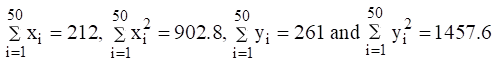Which is more variable, the length or weight?

Solution:

Compare the coefficients of variation (CV) to get required result.

Here the number of products are n = 50 for length and weight both.

Step 1: For length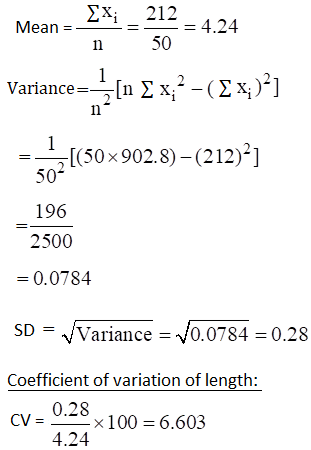Step 2: For weight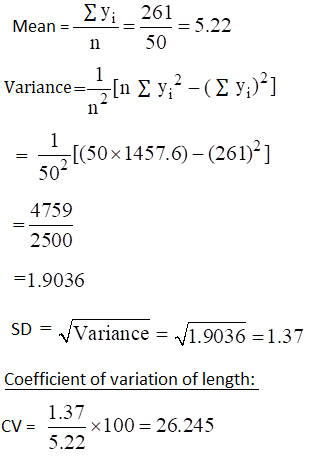From above results, we can say CV of weight > CV of length

Therefore, the weight is more variable than height.

## Access other exercise solutions of Class 11 Maths Chapter 30 Statistics

Exercise 30A Solutions

Exercise 30B Solutions

Exercise 30C Solutions